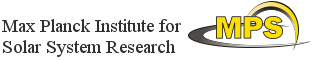## Exploitation of Space Data for Innovative Helio- and Asteroseismology

### Helioseismology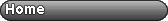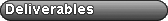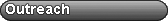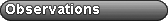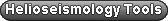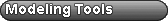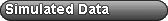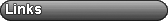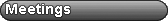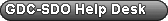# Fitting Travel Times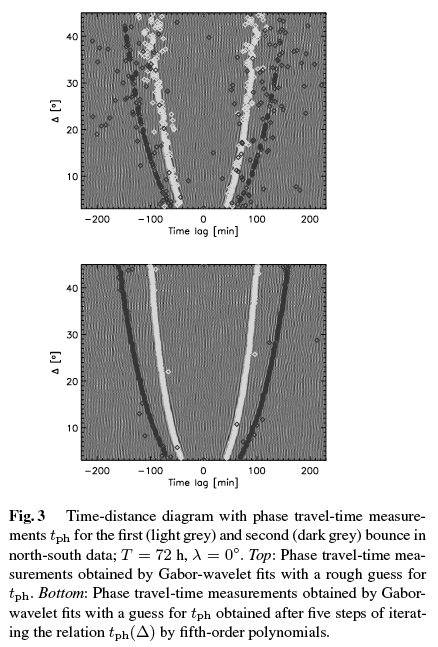The purpose of time-distance helioseismology is to calculate the travel time of solar waves between two surface points. The basic way to do this is to calculate the cross-covariance between the signal at the two points. The travel times are measured by fitting wavelets to the cross-covariance.

These IDL routines for measuring travel times from cross correlations. Two methods are implemented: a Gabor-wavelet fit and a one-parameter fit.
The input for each method are time-distance diagrams.
Included are example time-distance diagrams to use as input, and instructions on how to run the code.

Fitting travel times (tar file)

Documentation: Measuring helioseismic travel times (2007) M. Roth, L. Gizon, J.G. Beck, Astron. Nachr. 328, 215-222

webmaster